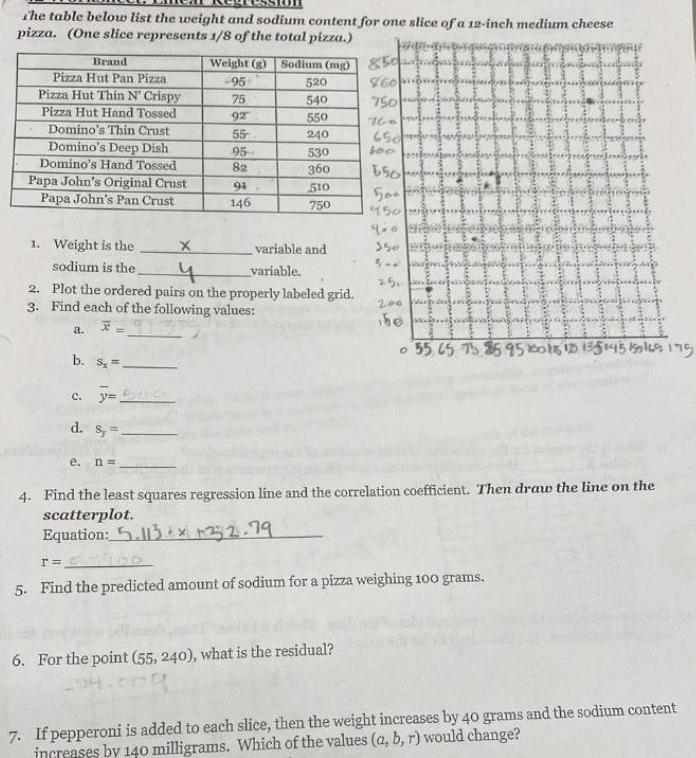Question:

# the table below list the weight and sodium content for one

Last updated: 3/10/2023the table below list the weight and sodium content for one slice of a 12 inch medium cheese pizza One slice represents 1 8 of the total pizza Wangs g Sodium mg 520 540 550 240 Brand Pizza Hut Pan Pizza Pizza Hut Thin N Crispy Pizza Hut Hand Tossed Domino s Thin Crust Domino s Deep Dish Domino s Hand Tossed Papa John s Original Crust Papa John s Pan Crust a b s c y c d S Weight 95 e n 75 92 55 95 82 1 Weight is the X sodium is the 4 2 Plot the ordered pairs on the properly labeled grid 3 Find each of the following values X 94 146 530 360 510 750 variable and variable 850 960 750 6 For the point 55 240 what is the residual 94 000 760 650 600 650 50 450 350 500 2 5 200 be prestavby X Sy Noki r 5 Find the predicted amount of sodium for a pizza weighing 100 grams sepert Swa Ring Berg F donarmiarknuddaterin autorow A Re Fax Foto E 55 65 75 95 95 200 115 125 135 145 155 165 175 4 Find the least squares regression line and the correlation coefficient Then draw the line on the scatterplot Equation 5 13x 32 79 7 If pepperoni is added to each slice then the weight increases by 40 grams and the sodium content increases by 140 milligrams Which of the values a b r would change